# 启发式算法 (Heuristic Algorithms)

1. 算法简单直观，易于修改
2. 算法能够在可接受的时间内给出一个较优解
1. 不能保证为全局最优解
2. 算法不稳定，性能取决于具体问题和设计者经验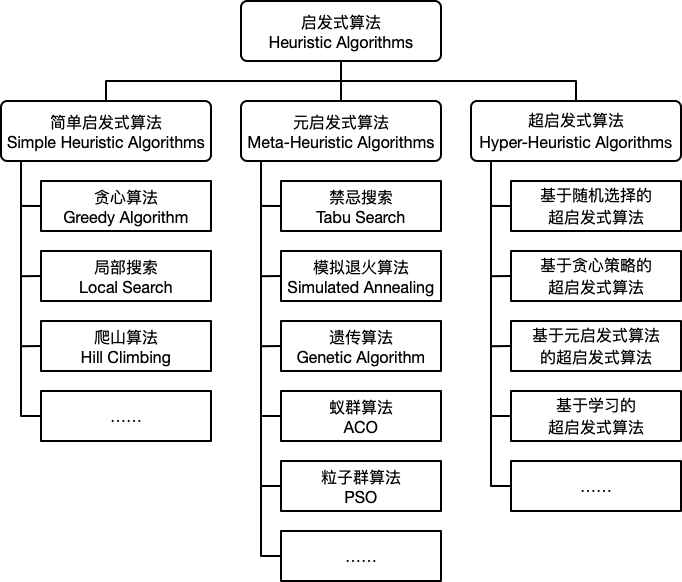# 简单启发式算法 (Simple Heuristic Algorithms)

## 贪心算法 (Greedy Algorithm)

1. 确定问题的最优子结构。
2. 设计递归解，并保证在任一阶段，最优选择之一总是贪心选择。
3. 实现基于贪心策略的递归算法，并转换成迭代算法。

1. 最优子结构性质。当一个问题具有最优子结构性质时，可用 动态规划 法求解，但有时用贪心算法求解会更加的简单有效。同时并非所有具有最优子结构性质的问题都可以利用贪心算法求解。
2. 贪心选择性质。所求问题的整体最优解可以通过一系列局部最优的选择 (即贪心选择) 来达到。这是贪心算法可行的基本要素，也是贪心算法与动态规划算法的主要区别。

## 局部搜索 (Local Search) 和爬山算法 (Hill Climbing)

$$\min f \left(x\right), x \in \mathbb{R}^n$$

1. 初始化一个可行解 $x$
2. 在当前解的邻域内选择一个移动后的解 $s \left(x\right)$，使得 $f \left(s \left(x\right)\right) < f \left(x\right), s \left(x\right) \in S \left(x\right)$，如果不存在这样的解，则 $x$ 为最优解，算法停止。
3. $x = s \left(x\right)$，重复步骤 2。

# 元启发式算法 (Meta-Heuristic Algorithms)

1. 给定一个初始可行解，将禁忌表设置为空。
2. 选择候选集中的最优解，若其满足渴望水平，则更新渴望水平和当前解；否则选择未被禁忌的最优解。
3. 更新禁忌表。
4. 判断是否满足停止条件，如果满足，则停止算法；否则转至步骤 2。

## 模拟退火 (Simulated Annealing)

1. 给定一个初始可行解 $x_0$，初始温度 $T_0$ 和终止温度 $T_f$，令迭代计数为 $k$
2. 随机选取一个邻域解 $x_k$，计算目标函数增量 $\Delta f = f \left(x_k\right) - f \left(x\right)$。若 $\Delta f < 0$，则令 $x = x_k$；否则生成随机数 $\xi = U \left(0, 1\right)$，若随机数小于转移概率 $P \left(\Delta f, T\right)$，则令 $x = x_k$
3. 降低温度 $T$
4. 若达到最大迭代次数 $k_{max}$ 或最低温度 $T_f$，则停止算法；否则转至步骤 2。

\begin{algorithm}
\caption{模拟退火算法}
\begin{algorithmic}
\STATE $x \gets x_0$
\STATE $T \gets T_0$
\STATE $k \gets 0$
\WHILE{$k \leq k_{max}$ \AND $T \geq T_f$}
\STATE $x_k \gets$ \CALL{neighbor}{$s$}
\STATE $\Delta f = f \left(x_k\right) - f \left(x\right)$
\IF{$\Delta f < 0$ \OR \CALL{random}{$0, 1$} $\leq P \left(\Delta f, T\right)$}
\STATE $x \gets x_k$
\ENDIF
\STATE $T \gets$ \CALL{cooling}{$T, k, k_{max}$}
\STATE $k \gets k + 1$
\ENDWHILE
\end{algorithmic}
\end{algorithm}


1. $T_{k+1} = T_k * r$，其中 $r \in \left(0.95, 0.99\right)$$r$ 设置的越大，温度下降越快。
2. $T_{k+1} = T_k - \Delta T$，其中 $\Delta T$ 为每一步温度的减少量。

$$f \left(x, y\right) = e^{- \left(x^2 + y^2\right)} + 2 e^{- \left(\left(x - 1.7\right)^2 + \left(y - 1.7\right)^2\right)}$$

$$\max f \left(x, y\right), x \in \left[-2, 4\right], y \in \left[-2, 4\right]$$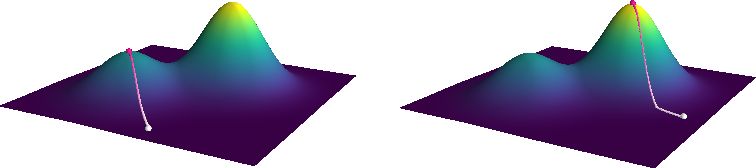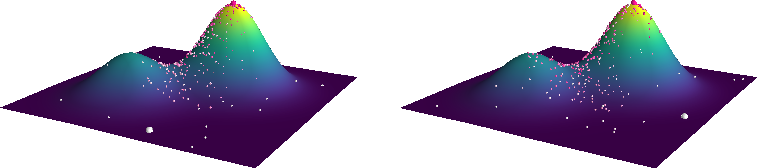## 遗传算法 (Genetic Algorithm)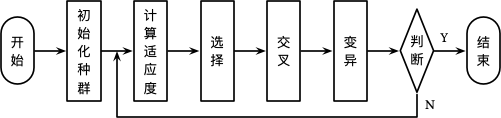### 初始化种群

$$x_i = 50_{10} = 0110010_{2}$$

$$x = \left(p_1, p_2, \dotsc, p_m\right)$$

### 选择

$$P_i = \dfrac{F_i}{\sum_{i=1}^{n}{F_i}}$$

$$\begin{equation} \begin{split} CP_0 &= 0 \\ CP_i &= \sum_{j=1}^{i}{P_i} \end{split} \end{equation}$$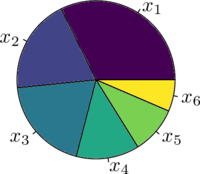### 交叉

• 单点交叉：在染色体中选择一个切点，然后将其中一部分同另一个染色体的对应部分进行交换得到两个新的个体。交叉过程如下图所示：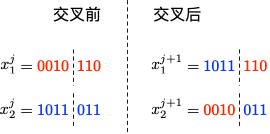• 多点交叉：在染色体中选择多个切点，对其任意两个切点之间部分以概率 $P_c$ 进行交换，其中 $P_c$ 为一个较大的值，例如 $P_m = 0.9$。两点交叉过程如下图所示：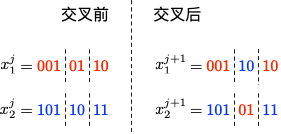• 均匀交叉：染色体任意对应的位置以一定的概率进行交换得到新的个体。交叉过程如下图所示：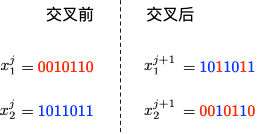### 小结

1. 选择：优胜劣汰，适者生存。
2. 交叉：丰富种群，持续优化。
3. 变异：随机扰动，避免局部最优。

### 示例 - 商旅问题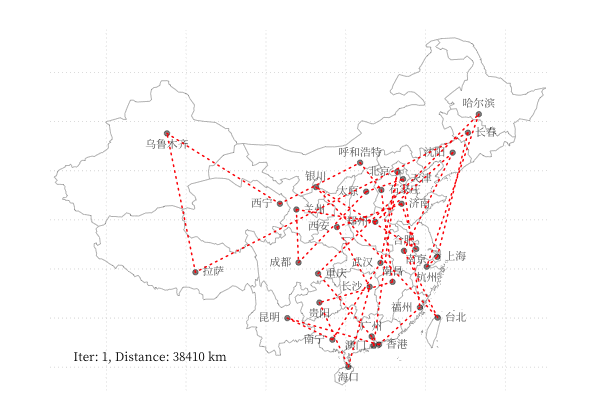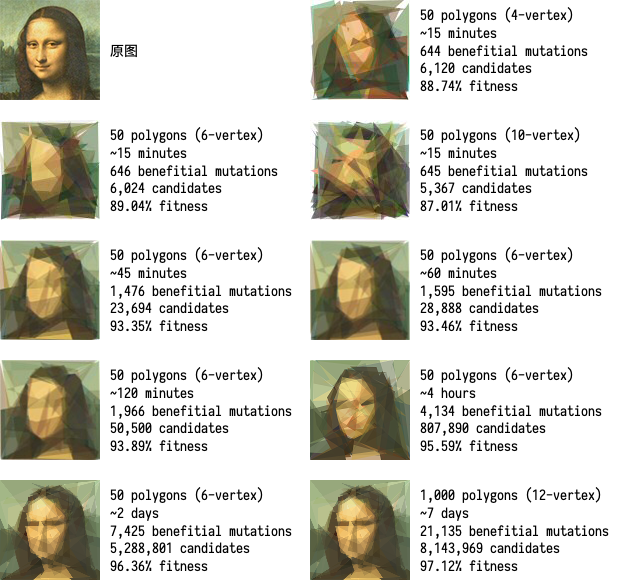## 蚁群算法 (Ant Colony Optimization, ACO)

1991 年，意大利学者 Dorigo M. 等人在第一届欧洲人工生命会议 (ECAL) 上首次提出了蚁群算法。1996 年 Dorigo M. 等人发表的文章 “Ant system: optimization by a colony of cooperating agents” 8 为蚁群算法奠定了基础。在自然界中，蚂蚁会分泌一种叫做信息素的化学物质，蚂蚁的许多行为受信息素的调控。蚂蚁在运动过程中能够感知其经过的路径上信息素的浓度，蚂蚁倾向朝着信息素浓度高的方向移动。以下图为例 9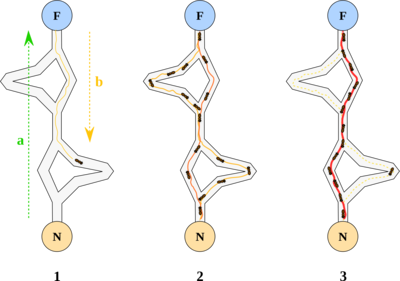## 粒子群算法 (Particle Swarm Optimization, PSO)

Eberhart, R. 和 Kennedy, J. 于 1995 年提出了粒子群优化算法 10 11。粒子群算法模仿的是自然界中鸟群和鱼群等群体的行为，其基本原理描述如下：

$$\begin{equation} \begin{split} v^{k+1}_i &= \omega v^k_i + c_1 \xi \left(p^k_i - x^k_i\right) + c_2 \eta \left(p^k_g - x^k_i\right) \\ x^{k+1}_i &= x^k_i + v^{k+1}_i \end{split} \end{equation}$$

$$f \left(x, y\right) = \left(1 - x\right)^2 + 100 \left(y - x^2\right)^2$$

$x \in \left[-2, 2\right], y \in \left[-1, 3\right]$，定义优化问题为最小化目标函数，最优解为 $\left(0, 0\right)$。利用 PySwarms 扩展包的优化过程可视化如下：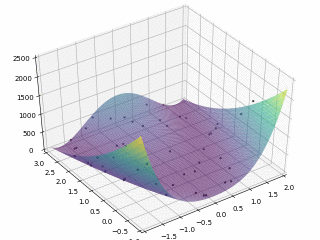# 超启发式算法 (Hyper-Heuristic Algorithms)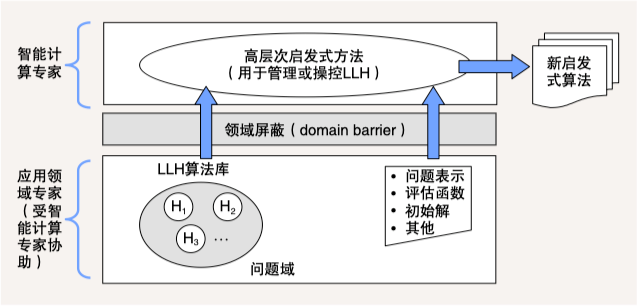1. 邢文训, & 谢金星. (2005). 现代优化计算方法. 清华大学出版社. ↩︎ ↩︎

2. 汪定伟, 王俊伟, 王洪峰, 张瑞友, & 郭哲. (2007). 智能优化方法. 高等教育出版社. ↩︎

3. Glover, F. W., & Laguna, M. (1997). Tabu Search. Springer US. ↩︎

4. Kirkpatrick, S., Gelatt, C. D., & Vecchi, M. P. (1983). Optimization by Simulated Annealing. Science, 220(4598), 671–680. ↩︎

5. Michalewicz, Z., Janikow, C. Z., & Krawczyk, J. B. (1992). A modified genetic algorithm for optimal control problems. Computers & Mathematics with Applications, 23(12), 83-94. ↩︎

6. Gottlieb, J., & Paulmann, L. (1998, May). Genetic algorithms for the fixed charge transportation problem. In Evolutionary Computation Proceedings, 1998. IEEE World Congress on Computational Intelligence., The 1998 IEEE International Conference on (pp. 330-335). IEEE. ↩︎

7. Dorigo, M., Maniezzo, V., & Colorni, A. (1996). Ant system: optimization by a colony of cooperating agents. IEEE Transactions on Systems, man, and cybernetics, Part B: Cybernetics, 26(1), 29-41. ↩︎

8. Toksari, M. D. (2016). A hybrid algorithm of Ant Colony Optimization (ACO) and Iterated Local Search (ILS) for estimating electricity domestic consumption: Case of Turkey. International Journal of Electrical Power & Energy Systems, 78, 776-782. ↩︎

9. Eberhart, R., & Kennedy, J. (1995, November). Particle swarm optimization. In Proceedings of the IEEE international conference on neural networks (Vol. 4, pp. 1942-1948). ↩︎

10. Eberhart, R., & Kennedy, J. (1995, October). A new optimizer using particle swarm theory. In MHS'95. Proceedings of the Sixth International Symposium on Micro Machine and Human Science (pp. 39-43). IEEE. ↩︎

11. 江贺. (2011). 超启发式算法：跨领域的问题求解模式. 中国计算机学会通讯, 7(2), 63-70 ↩︎